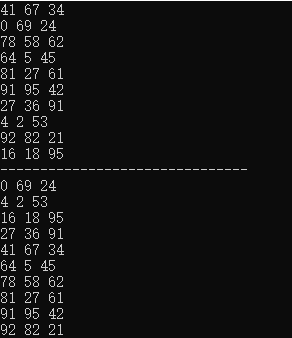• 题目描述 输入一颗二叉树的根节点和一个整数，打印出二叉树中...(注意: 在返回值的list中，数组长度大的数组靠前) /* struct TreeNode { int val; struct TreeNode *left; struct TreeNode *right; TreeNode(...
题目描述

输入一颗二叉树的根节点和一个整数，打印出二叉树中结点值的和为输入整数的所有路径。路径定义为从树的根结点开始往下一直到叶结点所经过的结点形成一条路径。(注意: 在返回值的list中，数组长度大的数组靠前)

带记忆的DFS

/*
struct TreeNode {
int val;
struct TreeNode *left;
struct TreeNode *right;
TreeNode(int x) :
val(x), left(NULL), right(NULL) {
}
};*/
class Solution {
vector<vector<int>> res;
vector<int> path;
void find(TreeNode* root,int sum){
//这两句可加可不加
if(sum < root->val)// 当前值不适合
return;
//路径输入
path.push_back(root->val);
//判断是否符合条件
if(!root->left && !root->right && sum == root->val)//到达叶子节点
res.push_back(path);
else{
if(root->left)//查找左子树
find(root->left,sum-root->val);
if(root->right)//查找右子树
find(root->right,sum-root->val);
}
//删除path的最后一个元素，它表示当前叶节点和根节点形成的路径不满足条件，
//删除叶节点，返回它的父节点
path.pop_back();
}
public:
vector<vector<int> > FindPath(TreeNode* root,int expectNumber) {
if(root==NULL)
return res;
find(root,expectNumber);//查找符合的路径
sort(res.begin(),res.end(),[](const vector<int> &a,const vector<int> &b) {return a.size()>b.size();});//降序排序
return res;
}
};

参考链接：

C++ sort vector<vector<int> > or vector<MyClass> 容器的排序

关于C++中vector和set使用sort方法进行排序

展开全文c++
• 二维数组中的查找信息卡片时间：2020-03-16题目：二维数组中的查找tag：search array题目描述在一个二维数组中（每个一维数组的长度相同），每一行都按照从左到右递增的顺序排序，每一列都按照从上到下递增的顺序...二维数组中的查找信息卡片时间：2020-03-16题目：二维数组中的查找tag：search array题目描述在一个二维数组中（每个一维数组的长度相同），每一行都按照从左到右递增的顺序排序，每一列都按照从上到下递增的顺序排序。请完成一个函数，输入这样的一个二维数组和一个整数，判断数组中是否含有该整数。解法一　暴力法解题思路这是最容易想到的，从左到右依次遍历整个二维数组，找到需要查找的数返回 true，没找到就返回 false。class Solution {
public:
bool Find(int target, vector<vector<int> > array) {
int row,col;
row=array.size();
col=array.size();
if(row==0||col==0)    //边界问题要考虑
return false;
for(int i=0;i<row;i++)
{
for(int j=0;j<col;j++)
{
if(array[i][j]==target)
return true;
}
}
return false;
}
};时间复杂度：O(M*N)(统一说明，Ｍ代表行数，Ｎ代表列数，下同)空间复杂度：O(1)解法二　左下／右上移动法解题思路利用二维数组的性质，每一行都按照从左到右递增的顺序排序，每一列都按照从上到下递增的顺序排序。这里以右上移动为例，左下移动类似。二维数组右上角的数 m 是该行最大的数，是该列最小的数。每次将 m 和目标值 target 比较：当 m < target，由于 m 已经是该行最大的元素，想要更大只有从列考虑，取值下移一位。当 m > target，由于 m 已经是该列最小的元素，想要更小只有从行考虑，取值左移一位。当 m = target，找到该值，返回 true。public:
bool Find(int target, vector<vector<int> > array) {
int row,col;
row=array.size();
col=array.size();
if(row==0||col==0)
return false;
int i = 0;
int j = col-1;
while(i<row && j>=0){
if(array[i][j] < target){
i++;
}else if(array[i][j] > target){
j--;
}else{
return true;
}
}
return false;
}
};时间复杂度：O(M+N)空间复杂度：O(1)解法三　二分查找解题思路从第一行开始，对每一行进行二分查找。如果找到 target 则返回 true，没找到就移动到下一行进行二分查找，直到查找到最后一行。class Solution {
public:
bool Find(int target, vector<vector<int> > array) {
int row,col;
row=array.size();
col=array.size();
if(row==0||col==0)
return false;
for(int i=0;i<row;i++)
{
int low=0;
int high=col-1;
while(low<=high)
{
int mid=low+(high-low)/2;
if(target==array[i][mid])
{
return true;
}
else if(target<array[i][mid])
{
high=mid-1;
}
else
{
low=mid+1;
}
}
}
return false;
}
};时间复杂度：O(MlogN)空间复杂度：O(1)知识扩展1.题解中使用的是 C++ 的容器 vector 来操作数组，这种容器与平常使用的内置数组相比有什么优势呢？各自的应用场合是哪里？优势：vector 支持动态扩容，适用于数组长度不断改变的情况；内置数组什么都需要亲力亲为，经常会出现数组越界的情况，而 vector 可以使用迭代器避免；vector 支持直接拷贝和赋值，内置数组无法进行直接拷贝和赋值。劣势：vector 存储大量数据时效率比内置数组低，且在分配连续大量内存时可能会失败。2.题目已经给了一个特定顺序的数组，如何自己使用 vector 进行行或列排序？#include<stdio.h>
#include<algorithm>
#include<vector>
#include<stdlib.h>
#include<iostream>
using namespace std;

int main()
{
vector<vector<int>> viA(10);
for (int i = 0; i < 10;i++)
for (int j = 0; j < 10; j++){
viA[i].push_back(rand()%100);
}
for (int i = 0; i < 10; i++){
for (int j = 0; j < 10; j++){
cout << viA[i][j] << "t";
}
cout << endl;
}
cout << "按行排序后的输出" << endl;
for (int i = 0; i < 10; i++){
sort(viA[i].begin(), viA[i].end());//默认为从小到大排序
}
for (int i = 0; i < 10; i++){
for (int j = 0; j < 10; j++){
cout << viA[i][j] << "t";
}
cout << endl;
}
while (1);
return 0;
}总结1.在数据量很大的情况下，三种常见解法的时间复杂度相比，解法二 < 解法三 < 解法一，换句话说，解法二最好，解法三次之，解法三最差。2.在做题的时候优先考虑边界问题，这样能更好地把问题考虑清楚。最后如果觉得手机端查看不方便，请点击阅读原文，里面有我的对应的 Github 项目，项目会持续更新，欢迎 Star，每一个 Star 都是最好的支持。
展开全文二维vector赋值
• 在一个二维数组中（每个一维数组的长度相同），每一行都按照从左到右递增的顺序排序，每一列都按照从上到下递增的顺序排序。请完成一个函数，输入这样的一个二维数组和一个整数，判断数组中是否含有该整数。 class ...
在一个二维数组中（每个一维数组的长度相同），每一行都按照从左到右递增的顺序排序，每一列都按照从上到下递增的顺序排序。请完成一个函数，输入这样的一个二维数组和一个整数，判断数组中是否含有该整数。
class Solution {
public:
bool Find(int target, vector
展开全文• 1.sort()函数，默认的是对二维数组按照第一列的大小对每行的数组进行排序。默认从小到大 #include <iostream> #include<vector> #include<algorithm> using namespace std; int main() { vector...
1.sort()函数，默认的是对二维数组按照第一列的大小对每行的数组进行排序。默认从小到大
#include <iostream>
#include<vector>
#include<algorithm>
using namespace std;

int main()
{
vector<vector<int> > intervals(10);
for (int i = 0; i < 10; i++)
{
for (int j = 0; j < 3; j++)
{
intervals[i].push_back(rand() % 100);
}
}
for (int i = 0; i < 10; i++)
{
for (int j = 0; j < 3; j++)
{
cout<<intervals[i][j]<<" ";
}
cout<<endl;
}
sort(intervals.begin(),intervals.end());
cout<<"-------------------------------"<<endl;
for (int i = 0; i < 10; i++)
{
for (int j = 0; j < 3; j++)
{
cout<<intervals[i][j]<<" ";
}
cout<<endl;
}
return 0;
}


结果：自定义比较函数：按照第一列从大到小，如果相等则按照第二列从小到大
bool cmp(vector<int>&a,vector<int>&b){
if(a!=b) return a>b;
else return a<b;
}
sort(intervals.begin(),intervals.end(),cmp);

完整代码：
#include <iostream>
#include<vector>
#include<algorithm>
using namespace std;
bool cmp(vector<int>&a,vector<int>&b){
if(a!=b) return a>b;
else return a<b;
}
int main()
{
vector<vector<int> > intervals(10);
for (int i = 0; i < 10; i++)
{
for (int j = 0; j < 3; j++)
{
intervals[i].push_back(rand() % 100);
}
}
for (int i = 0; i < 10; i++)
{
for (int j = 0; j < 3; j++)
{
cout<<intervals[i][j]<<" ";
}
cout<<endl;
}
sort(intervals.begin(),intervals.end(),cmp);
cout<<"-------------------------------"<<endl;
for (int i = 0; i < 10; i++)
{
for (int j = 0; j < 3; j++)
{
cout<<intervals[i][j]<<" ";
}
cout<<endl;
}
return 0;
}


或者写作：
sort(intervals.begin(),intervals.end(),[](const vector<int>& a,const vector<int>& b){
return  a<b;
});



展开全文• 在一个二维数组中，每一行都按照从左到右递增的顺序排序，每一列都按照从上到下递增的顺序排序。请完成一个函数，输入这样的一个二维数组和一个整数，判断数组中是否含有该整数。 解题思路： 第一行到最后一行是...剑指offer
• 写一个bool类型的comp函数，比如下面根据第个元素排序： bool cmp1(const vector<int> &a, const vector<int> &b){ return a > b; } sort(allvec.begin(), allvec.end(), cmp1...
• 在一个二维数组中（每个一维数组的长度相同），每一行都按照从左到右递增的顺序排序，每一列都按照从上到下递增的顺序排序。请完成一个函数，输入这样的一个二维数组和一个整数，判断数组中是否含有该整数。 #...
• 在一个 n * m的二维数组中，每一行都按照从左到右递增的顺序排序，每一列都按照从上到下递增的顺序排序。请完成一个高效的函数，输入这样的一个二维数组和一个整数，判断数组中是否含有该整数。 一、解题思路 从...
• 4.vector 二维数组的push_back vector&amp;amp;lt;vector&amp;amp;lt;int&amp;amp;gt; &amp;amp;gt;vec; vector&amp;amp;lt;int&amp;amp;gt;array1; for (int i=0;i&amp;amp;lt;num...
• 参考：使用vector创建二维动态数组，并使用sort对其进行排序 程序首先构建二维vector数组viA，然后对其进行打印，之后，按照不同的方式对其进行排序。 程序如下： #include <iostream> #include <vector>...
• class Solution { ...static bool cmp(vector<int> &a, vector<int> &b) {return a<b;} int findLongestChain(vector<vector<int>>& pairs) { if(p...
• 最后研究的结果：不用vector排序了，直接自定义结构体(在c++中是结构体struct，Java 中...https://mp.csdn.net/postedit/88592611 这个是我最终写的java实现二维数组排序，我觉得还是自己写得好理解，实用。下面是我...
• 最后研究的结果：不用vector排序了，直接自定义结构体（在c++中是结构体struct，Java 中是...https://mp.csdn.net/postedit/88592611 这个是我最终写的java实现二维数组排序，我觉得还是自己写得好理解，实用。 ...
• 对于一维数组可以方便的使用sort进行排序，那么如何用sort对二维数组进行排序呢？只需要自定义比较方法即可。 vector<vector<int> > demo; sort(demo.begin(),demo.end(),[](vector<int> a,...数据结构 c++...